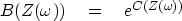Next: Finding a causal wavelet Up: SPECTRAL FACTORIZATION Previous: SPECTRAL FACTORIZATION

## The exponential of a causal is causal.

Begin with a causal response ct and its associated C(Z). The Z-transform C(Z) could be evaluated, giving a complex value for each real.This complex value could be exponentiated to get another value, say(15)
Next, we could inverse transformback to bt. We will prove the amazing fact that bt must be causal too.

First notice that if C(Z) has no negative powers of Z, then C(Z)2 does not either. Likewise for the third power or any positive integer power, or sum of positive integer powers. Now recall the basic power-series definition of the exponential function:(16)
Including equation (15) gives the exponential of a causal:(17)
Each term in the infinite series corresponds to a causal response, so the sum, bt, is causal. (If you have forgotten the series for the exponential function, then recall that the solution to dy/dx=y is the definition of the exponential function y(x)=ex, and that the power series satisfies the differential equation term by term, so it must be the exponential too. The factorials in the denominators assure us that the power series always converges, i.e., it is finite for any finite x.)

Putting one polynomial into another or one infinite series into another is an onerous task, even if it does lead to a wavelet that is exactly causal. In practice we do operations that are conceptually the same, but for speed we do them with discrete Fourier transforms. The disadvantage is periodicity, i.e., negative times are represented computationally like negative frequencies. Negative times are the last half of the elements of a vector, so there can be some blurring of late times into negative ones.

 eZ Figure 9 Exponentials.Figure 9 gives examples of equation (17) for C=Z and C=4Z. Unfortunately, I do not have an analytic calculation to confirm the validity of these examples. 1Next: Finding a causal wavelet Up: SPECTRAL FACTORIZATION Previous: SPECTRAL FACTORIZATION
Stanford Exploration Project
10/21/1998